# Layer1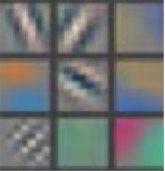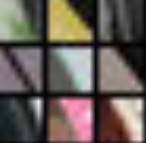# Layer2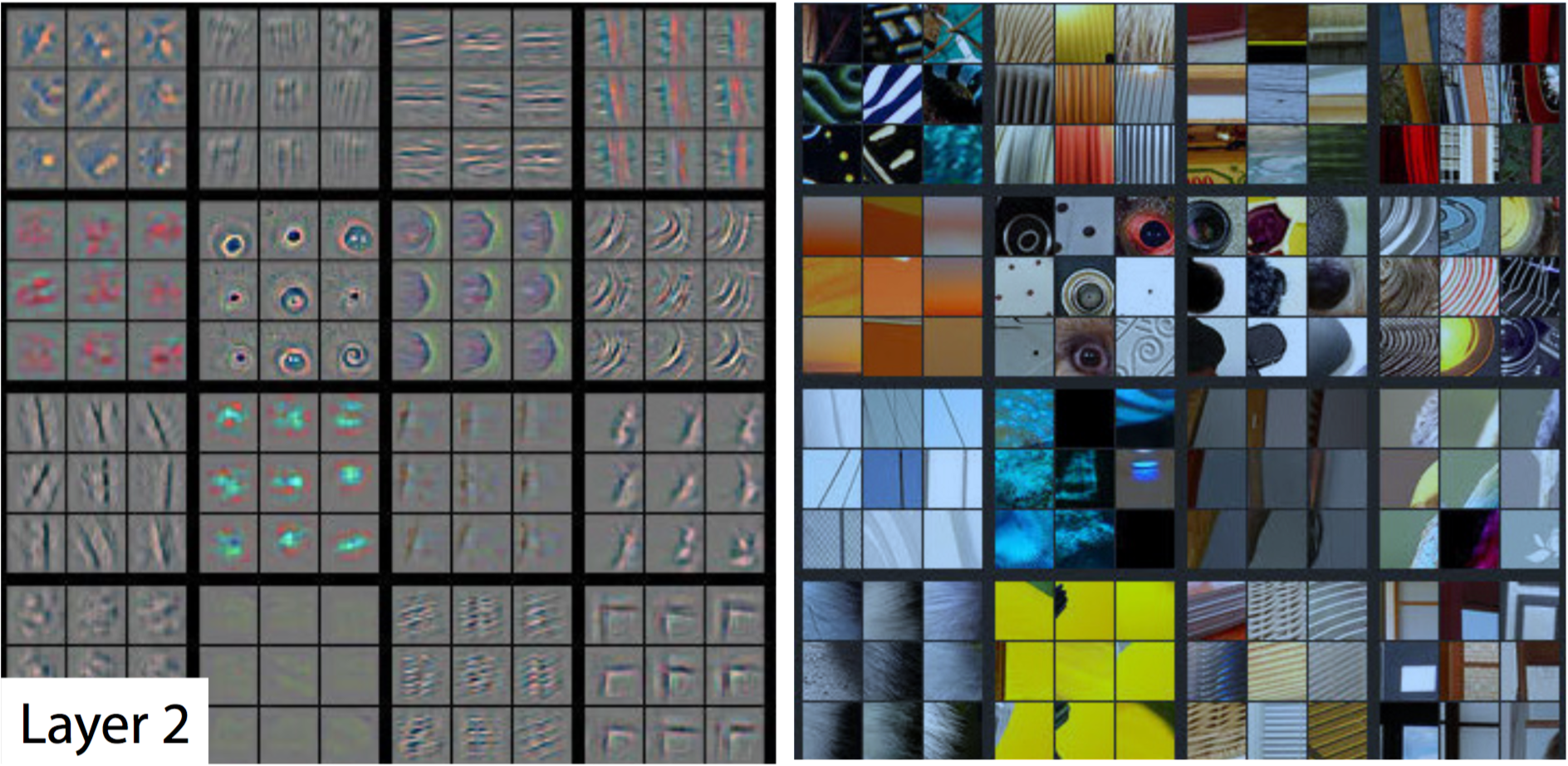# Layer3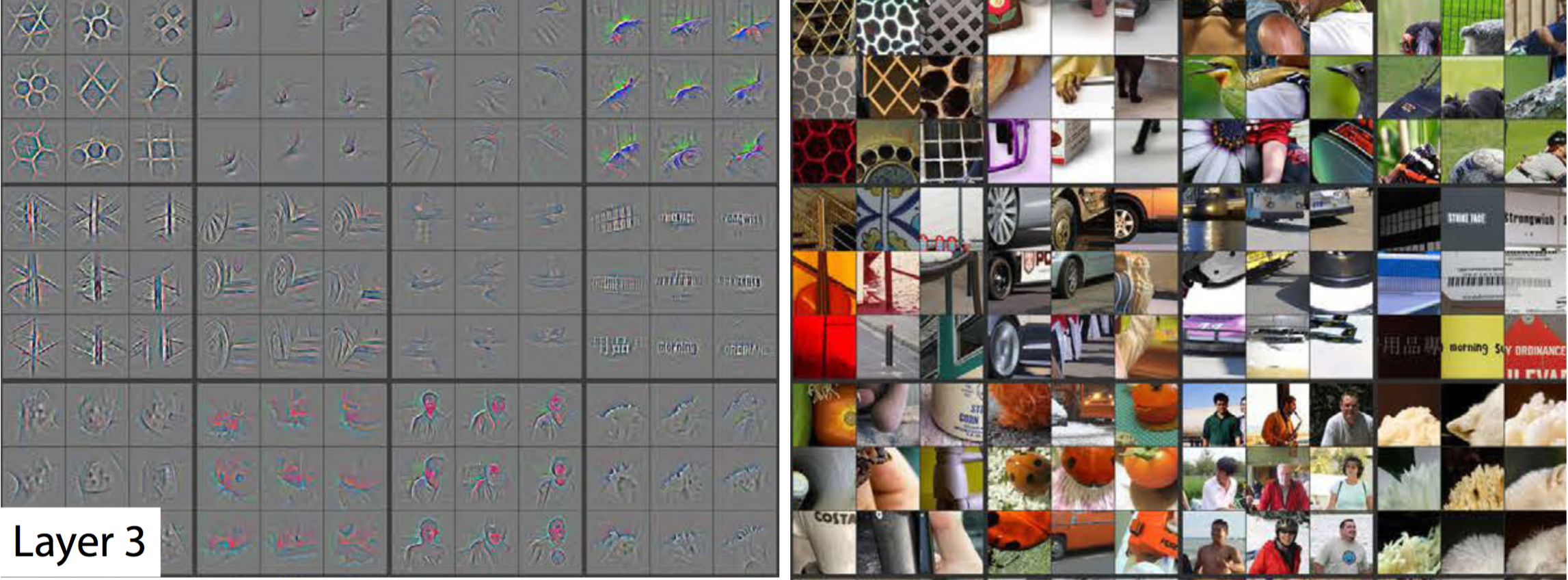# Layer5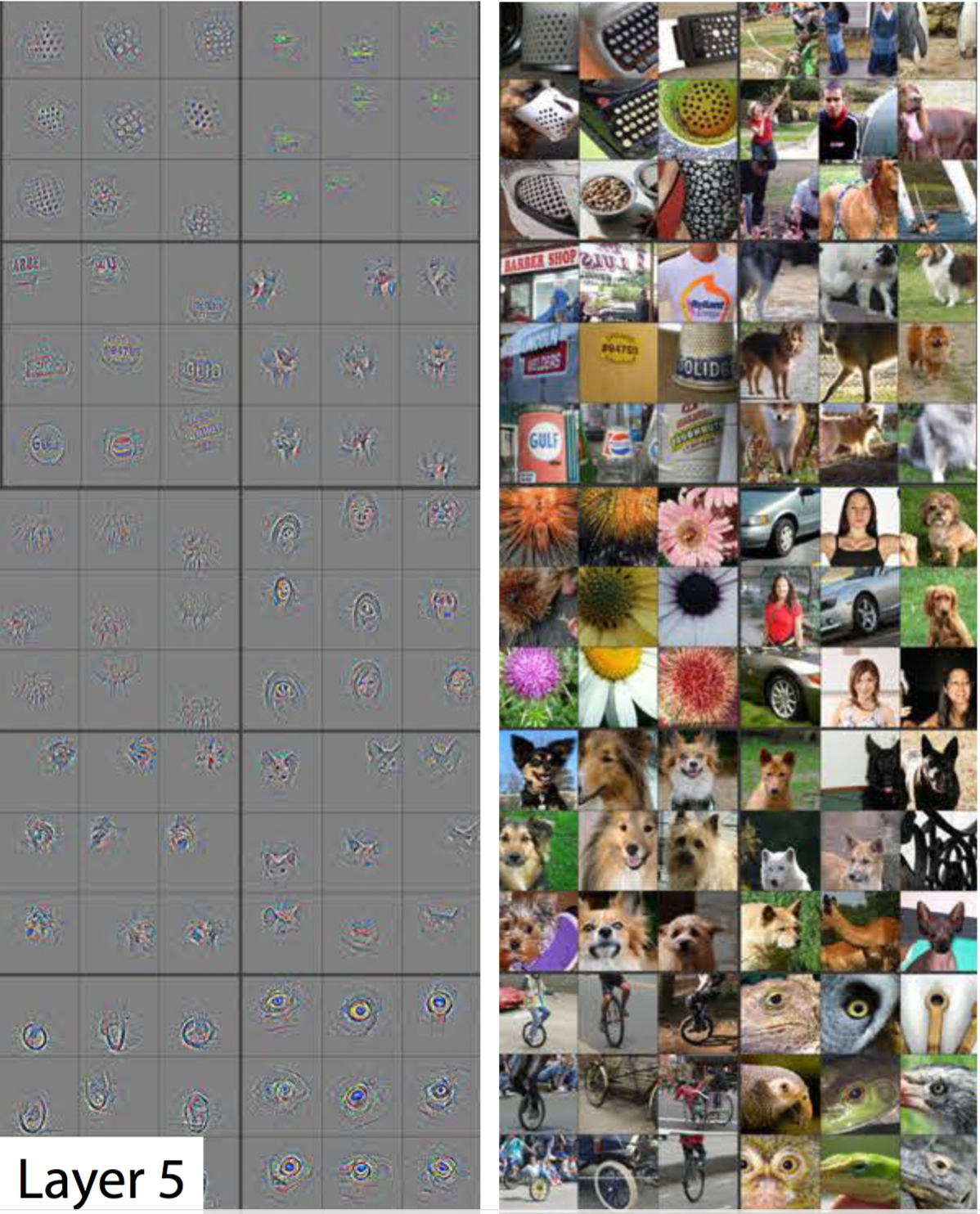# 在TensorFlow中使用CNN

``````# Output depth
k_output = 64

# Image Properties
image_width = 10
image_height = 10
color_channels = 3

# Convolution filter
filter_size_width = 5
filter_size_height = 5

# Input/Image
input = tf.placeholder(
tf.float32,
shape=[None, image_height, image_width, color_channels])

# Weight and bias
weight = tf.Variable(tf.truncated_normal(
[filter_size_height, filter_size_width, color_channels, k_output]))
bias = tf.Variable(tf.zeros(k_output))

# Apply Convolution
conv_layer = tf.nn.conv2d(input, weight, strides=[1, 2, 2, 1], padding='SAME')
# Apply activation function
conv_layer = tf.nn.relu(conv_layer)
``````

Github链接

# 更多文章请关注我的个人网站：

weiweizhao.com

0 回复

### 发表评论

Want to join the discussion?
Feel free to contribute!